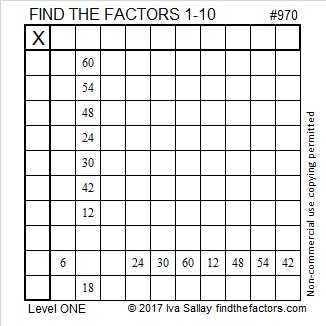# 970 and Level 1

This level 1 puzzle will help you focus on one set of division facts. You can find all the factors that belong in the first column and the top row if you know those division facts. After you find all the factors from 1 to 10, you can fill in the entire multiplication table.Print the puzzles or type the solution in this excel file: 10-factors-968-977

970 is the sum of two squares two different ways:
23² + 21² = 970
31² + 3²= 970

That means 970 is the hypotenuse of more than one Pythagorean triple:
88-966-970 calculated from 23² – 21², 2(23)(21), 23² + 21²
186-952-970 calculated from 2(31)(3), 31² – 3², 31² + 3²
582-776-970 which is (3-4-5) times 194
650-720-970 which is 10 times (65-72-97)

Here’s a fun fact: 970 is 202 in BASE 22 because 2(22²) + 2(1) = 2(484 + 1) = 2(485) = 970

• 970 is a composite number.
• Prime factorization: 970 = 2 × 5 × 97
• The exponents in the prime factorization are 1, 1, and 1. Adding one to each and multiplying we get (1 + 1)(1 + 1)(1 + 1) = 2 × 2 × 2 = 8. Therefore 970 has exactly 8 factors.
• Factors of 970: 1, 2, 5, 10, 97, 194, 485, 970
• Factor pairs: 970 = 1 × 970, 2 × 485, 5 × 194, or 10 × 97
• 970 has no square factors that allow its square root to be simplified. √970 ≈ 31.14482This site uses Akismet to reduce spam. Learn how your comment data is processed.